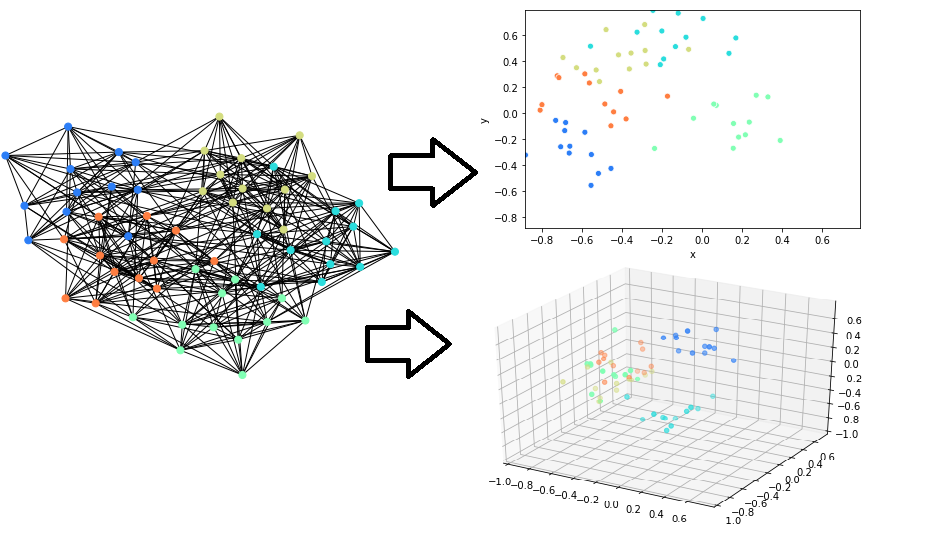This package implements fast/scalable node embedding algorithms. This can be used to embed the nodes in graph objects. We support NetworkX graph types natively.

You can also efficiently embed arbitrary scipy CSR Sparse Matrices, though not all algorithms here are optimized for this usecase.## Installing

`pip install nodevectors`

## Supported Algorithms

• GGVec (paper upcoming)

• GLoVe (paper). This is useful to embed word co-occurence in sparse matrix form.

• Any Scikit-Learn API model that supports the `fit_transform` method with the `n_component` attribute (eg. all manifold learning models, UMAP, etc.). Used with the `SKLearnEmbedder` object.

## Quick Example:

``````    import networkx as nx
from nodevectors import Node2Vec

# Test Graph
G = nx.generators.classic.wheel_graph(100)

# Fit embedding model to graph
g2v = Node2Vec()
# way faster than other node2vec implementations
# Graph edge weights are handled automatically
g2v.fit(G)

# query embeddings for node 42
g2v.predict(42)

# Save and load whole node2vec model
# Uses a smart pickling method to avoid serialization errors
g2v.save('node2vec.pckl')

# Save model to gensim.KeyedVector format
g2v.save_vectors("wheel_model.bin")

from gensim.models import KeyedVectors
model[str(43)] # need to make nodeID a str for gensim
``````

Warning: Saving in Gensim format is only supported for the Node2Vec model at this point. Other models build a `Dict` or embeddings.

## Embedding a large graph

NetworkX doesn't support large graphs (>500,000 nodes) because it uses lots of memory for each node. We recommend using CSRGraphs (which is installed with this package) to load the graph in memory:

``````import csrgraph as cg
import nodevectors

ggvec_model = nodevectors.GGVec()
embeddings = ggvec_model.fit_transform(G)``````

The ProNE and GGVec algorithms are the fastest. GGVec uses the least RAM to embed larger graphs. Additionally here are some recommendations:

• Don't use the `return_weight` and `neighbor_weight` if you are using the Node2Vec algorithm. It necessarily makes the walk generation step 40x-100x slower.

• If you are using GGVec, keep `order` at 1. Using higher order embeddings will take quadratically more time. Additionally, keep `negative_ratio` low (~0.05-0.1), `learning_rate` high (~0.1), and use aggressive early stopping values. GGVec generally only needs a few (less than 100) epochs to get most of the embedding quality you need.

• If you are using ProNE, keep the `step` parameter low.

• If you are using GraRep, keep the default embedder (TruncatedSVD) and keep the order low (1 or 2 at most).

## Embedding a VERY LARGE graph

(Upcoming).

GGVec can be used to learn embeddings directly from an edgelist file (or stream) when the `order` parameter is constrained to be 1. This means you can embed arbitrarily large graphs without ever loading them entirely into RAM.

### Related Projects

• GraphVite is not a python package but aims to have some of the more scalable embedding algorithm implementations.

• KarateClub Is specifically to embed NetworkX graphs. The implementations are less scalable, but the package is more complete and has more types of embedding algorithms.

## Why is it so fast?

We leverage CSRGraphs for most algorithms. This uses CSR graph representations and a lot of Numba JIT'ed procedures.